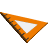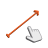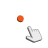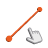You are here: Start » AVL.NET » Geometry 2D Interpolations

Geometry 2D Interpolations

IconNameDescriptionAveragePointComputes the middle point of two input points.LerpAnglesLinearly interpolates between two angles in the direction of minimum turn.LerpPathsLinearly interpolates between two paths.LerpPointsLinearly interpolates between two points.LerpSegmentsLinearly interpolates between two segments.LerpVectorsLinearly interpolates between two vectors.LerpVectors_RadialLinearly interpolates between two radial vectors.MedianAngleComputes the median angle of the input angles.